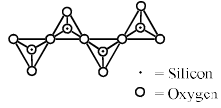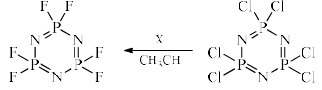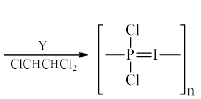# Test: Main Group - 1

## 30 Questions MCQ Test Inorganic Chemistry | Test: Main Group - 1

Description
Attempt Test: Main Group - 1 | 30 questions in 90 minutes | Mock test for Chemistry preparation | Free important questions MCQ to study Inorganic Chemistry for Chemistry Exam | Download free PDF with solutions
QUESTION: 1

Solution:
QUESTION: 2

### Which one of the following order of the carbonates is correct for their decomposition temperature:

Solution:
*Multiple options can be correct
QUESTION: 3

### The common feature (s) of Rb+, Kr and Br– is /are that they:

Solution:
*Multiple options can be correct
QUESTION: 4

The characteristics of the blue solution of solution of sodium in liquid ammonia is/are:

Solution:
QUESTION: 5

Do you expect the minimum energy necessary to eject a 3s electron from phosphorus in a photoelectron spectroscopy experiment for the process.

[Ne] 3s23p3 → [Ne] 3s13p3 + e- ,

Be larger than, smaller than, or the same as the 4th io nization energy (IE4) of phosphorus:

Solution:

Due to better guarding of s electrons by p if we directly remove an s electron without removing the p electrons, it would take more energy had we removed all outer electrons before.

Hence A is correct.

QUESTION: 6

Which of the following will be strongest acid in pure liquid HF:

Solution:

Explanation : Fluoroantimonic acid is the strongest superacid based on the measured value of its Hammett acidity function (H0), which has been determined for different ratios of HF:SbF5. While the H0 of pure HF is −15, addition of just 1 mol % of SbF5 lowers it to around −20.

QUESTION: 7

What is the nature of silicon–oxygen bonds in silica(SiO2):

Solution:
QUESTION: 8

The melting point of lithium metal is 454 K, and that of sodium is 371 K. Which is the following statements can explain this difference in their melt ing points:

(I) Metallic bonding in lithium is stronger than metallic bonding in sodium.
(II) The delocalized electrons are more strongly attracted to the metal cation of lithium.
(III) The lithium cations have a greater charge density than sodium cation.
(IV) Li+ cations are smaller than Na+ cations.

Solution:
QUESTION: 9

Although both NF3 and NCl3 are covalent, NCl3 undergoes hydrolysis whereas NF3 does not because:

Solution:
QUESTION: 10

The Lewis acidity of BF3 is less than BCl3 even though fluorine is more electronegative than chlorine. It is due to:

Solution:
QUESTION: 11

Pyroenes are a class of silicate minerals, which exhibit a polymeric chain structure, as shown below:Its simplest repeat unit is

Solution:
QUESTION: 12

In the following reactions:The reagent/conditions X and Y are:

Solution:

Hexachlorophosphazene gives Sn2 reaction with NaF and heating hexachlorophosphazene to  250 °C induces polymerization.

QUESTION: 13

Among the following, the group of molecules that undergoes rapid hydrolysis is:

Solution:
QUESTION: 14

The reaction of so lid XeF2 with AsF5 in 1:1 ratio affords:

Solution:
QUESTION: 15

For Et2AlX (X = PPh2, Ph, Cl– –and H), the tendency towards dimeric structure fo llows the order:

Solution:
QUESTION: 16

Na2HPO4 and NaH2PO4 on heating at high temperature produce a chain sodium pentaphosphate quantitatively.

Q.

The ideal molar ratio of Na2HPO4 to NaH2PO4 is:

Solution:

2Na2HPo4 + 3NaH2Po4 --->Na7P5o16

QUESTION: 17

Na2HPO4 and NaH2PO4 on heating at high temperature produce a chain sodium pentaphosphate quantitatively.

Q.

The total charge on pentaphosphate anion is:

Solution:

Explanation : Sodium tripolyphosphate is produced by heating a stoichiometric mixture of disodium phosphate, Na2HPO4, and monosodium phosphate, NaH2PO4, under carefully controlled conditions.

2Na2HPO4 + NaH2PO4 → Na5P3O10 + 2 H2O

STPP is a colourless salt, which exists both in anhydrous form and as the hexahydrate. The anion can be described as the pentanionic chain [O3POP(O)2OPO3]5-

QUESTION: 18

Among the following substituted silanes, the one that gives cross–linked silicone polymer upon hydrolysis is:

Solution:
QUESTION: 19

Among the following donors, the one that forms most stable adduct with the Lewis acid B(CH3)3 is:

Solution:
QUESTION: 20

The IUPAC nomenclature on Na[PCl6] is:

Solution:
QUESTION: 21

If a mixture of NaCl, conc. H2SO4 and K2Cr2O7 is heated in a dry test tube, a red vapour (P) is formed. This vapour (P) dissolves in aqueous NaOH to form a yellow solution, which upon treatment with AgNO3 forms a red solid (Q). P and Q are, respectively:

Solution:
QUESTION: 22

Heating a mixture of ammonium chloride and sodium tetrahydridoborate gives one liquid product(X), along with other products under ambient conditions.

NH4Cl + NaBH4 ------------> X + H+ NaCl

Q.

Compound X is:

Solution:

NH4Cl + NaBH4 ------------> B3N3H+ H+ NaCl

QUESTION: 23

Heating a mixture of ammonium chloride and sodium tetrahydridoborate gives one liquid product(X), along with other products under ambient conditions.

NH4Cl + NaBH4 ------------> X + H+ NaCl

Q.

Compound X is an example of:

Solution:
QUESTION: 24

An example of nido–borane from the following is:

Solution:
*Answer can only contain numeric values
QUESTION: 25

The number of S–S bonds in H2S5O6 is

Solution:
QUESTION: 26

The ease of formation of the adduct, NH3 · BX3 (where X = F, Cl, Br) follows the order:

Solution:
QUESTION: 27

Ammonolysis of S2Cl2 in an inert solvent gives:

Solution:
QUESTION: 28

Using Wade’s  rule predict the structure of B5H9:

Solution:
QUESTION: 29

Which among the following is a “superoxide”:

Solution:
QUESTION: 30

Aqua regia is a powerful oxidizing agent because it contains:

Solution:Use Code STAYHOME200 and get INR 200 additional OFF Use Coupon Code